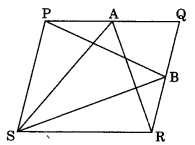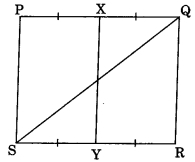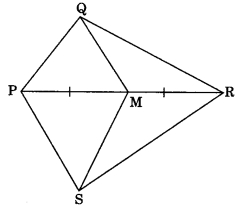# CBSEtips.in

## Saturday, 20 February 2021

### CBSE Class 9 Maths - MCQ and Online Tests - Unit 9 - Areas of Parallelograms and Triangles

#### CBSE Class 9 Maths – MCQ and Online Tests – Unit 9 – Areas of Parallelograms and Triangles

Every year CBSE  schools conducts Annual Assessment exams for 6,7,8,9,11th standards. These exams are very competitive to all the students. So our website provides online tests for all the 6,7,8,9,11th standard’s subjects. These tests are also very effective and useful for those who preparing for any competitive exams like Olympiad etc. It can boost their preparation level and confidence level by attempting these chapter wise online tests.

These online tests are based on latest CBSE syllabus. While attempting these our students can identify the weak lessons and continuously practice those lessons for attaining high marks. It also helps to revise the NCERT textbooks thoroughly.

#### CBSE Class 9 Maths – MCQ and Online Tests – Unit 9 – Areas of Parallelograms and Triangles

Question 1.
A, B, C and D are the mid-points of sides of parallelogram PQRS. If ar(PQRS) = 36 cm², then ar(ABCD) is(a) 24 cm²
(b) 18 cm²
(c) 30 cm²
(d) 36 cm²

Answer: (b) 18 cm²

Question 2.
If a triangle and a parallelogram are on the same base and between same parallels, then the ratio of the area of the triangle to the area of parallelogram is
(a) 1 : 3
(b) 1 : 2
(c) 3 : 1
(d) 1 : 4

Answer: (b) 1 : 2

Question 3.
The median of a triangle divides it into two
(a) isosceles triangle
(b) congruent triangles
(c) right angled triangle
(d) triangles of equal areas

Answer: (d) triangles of equal areas

Question 4.
PQRS is a parallelogram and A and B are any points on PQ and QR. If ar(PQRS) = 48 cm², then ar(?PBS) + ar(?ASR) is equal to(a) 96 cm²
(b) 36 cm²
(c) 48 cm²
(d) 24 cm²

Answer: (c) 48 cm²

Question 5.
D and E are the mid-points of BC and AD respectively. If ar(?ABC) = 12 cm², then ar(?BDE) is
(a) 5 cm²
(b) 6 cm²
(c) 3 cm²
(d) 9 cm²

Answer: (c) 3 cm²

Question 6.
ABCD is a quadrilateral whose diagonal AC divides it in two parts of equal area, then ABCD is a
(a) rectangle
(b) rhombus
(c) parallelogram
(d) need not be any of (a), (b) or (c)

Answer: (d) need not be any of (a), (b) or (c)

Question 7.
Two parallelograms are on equal bases and between the same parallels. The ratio of their areas is
(a) 1 : 2
(b) 1 : 1
(c) 2 : 1
(d) 3 : 1

Answer: (b) 1 : 1

Question 8.
PQRS is a parallelogram. If X and Y are the mid-points of PQ and SR and diagonal SQ is joined, then ar(XQRY) : ar(?QSR) is(a) 1 : 2
(b) 1 : 4
(c) 1 : 1
(d) 2 : 1

Answer: (c) 1 : 1

Question 9.
In quadrilateral PQRS, M is the mid-point of PR. If ar(SMQR) = 18 cm², then ar(PQMS) is(a) 24 cm²
(b) 12 cm²
(c) 18 cm²
(d) 36 cm²

Answer: (c) 18 cm²

Question 10.
ABCD is a trapezium in which AB || DC. If ar(?ABD) = 24 cm² and AB = 8 cm, then height of ?ABC is(a) 3 cm
(b) 6 cm
(c) 8 cm
(d) 4 cm

Answer: (d) 4 cm

Share: